## Tuesday, November 11, 2008

### Irodov Problem 1.239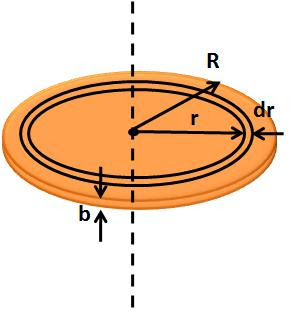a) Let the density of the disc be p. Consider a infinitesimally thin slice of the disc in the shape of a ring of thickness dr and width b -same as that of the disc. The mass of this ring will be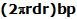. The reason we chose this particular shape (ring) for the infinitesimally thin ring is because all points of this ring are equidistant from the axis of rotation being at a distance r.
The moment of inertia can now be calculated as,b)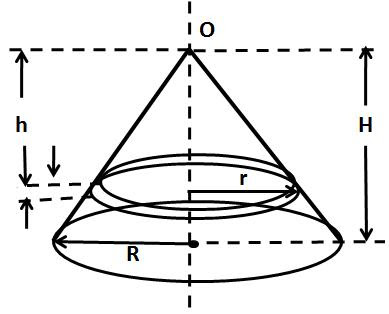We have already determined the moment of inertia of a disc of radius r and thickness b in the part a) of the problem. A cone is simply a stack of several infinitesimally thin disks of gradually decreasing radii, stack on top of one another. Consider an infinitesimally thin disc of thickness dh, at a distance h from the apex of the cone O. Let the height of the cone be H. The radius of this infinitesimally thick disc will be. Let the density of the cone be p. Based on our solution in part a), the moment of intertia of this infinitesimally thin disc will be,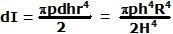The total moment of inertia of the entire cone can be determined by integrating (summing) over all infinitesimally thin discs as,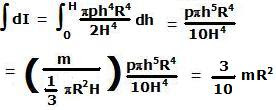Isn't it interesting to see that the moment of inertia of a cone does not depend on its height but only on the radius of its base!!